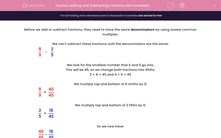# Adding and Subtracting Fractions with Unrelated Denominators

In this worksheet, students add and subtract fractions, where the denominator of one fraction is not a multiple of the other.Key stage:  KS 2

Curriculum topic:   Maths and Numerical Reasoning

Curriculum subtopic:   Fractions

Difficulty level:#### Worksheet Overview

Before we add or subtract fractions, they need to have the same denominators by using lowest common multiples.

We can't subtract these fractions until the denominators are the same:

 8 - 2 9 5

We look for the smallest number that 9 and 5 go into.

This will be 45, so we change both fractions into 45ths.

5 × 9 = 45 and 9 × 5 = 45

We multiply top and bottom of 8 ninths by 5:

 8 = 40 9 45

We multiply top and bottom of 2 fifths by 9:

 2 = 18 5 45

So we now have:

 40 - 18 45 45

 22 45

Example

Work out the following, remembering to give your answer in its lowest terms.

 2 + 3 3 4

The lowest common multiple of 3 and 4 is 12, because 4 × 3 = 3 × 4 = 12.

We multiply top and bottom of 2 thirds by 4:

 2 = 8 3 12

We multiply top and bottom of 3 quarters by 3:

 3 = 9 4 12

So we now have:

 8 + 9 12 12

This gives:

 17 12

But this must be changed to a mixed number.

17 ÷ 12 = 1 remainder 5.